• 今天小编就为大家分享一篇对Python中一维向量和一维向量转置相乘的方法详解，具有很好的参考价值，希望对大家有所帮助。一起跟随小编过来看看吧
• 运算 multiply （若x，y同为行/列向量，则简单的对应点对应相乘） multiply 运算每个数字对应相乘： 1）单纯列表 x = [1,2,3] y = [1,1,4] mul = multiply(x,y) print(type(mul)) print(mul) result： 2） array x ...
@TOCpython 矩阵向量乘积整理
运算 multiply （若x，y同为行/列向量，则简单的对应点对应相乘） multiply 运算每个数字对应相乘： 1）单纯列表 x = [1,2,3] y = [1,1,4] mul = multiply(x,y) print(type(mul)) print(mul)
result：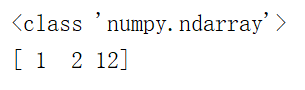2） array x = array([1,2,3]) y = array([1,1,4]) mul = multiply(x,y) print(type(mul)) print(mul)
result：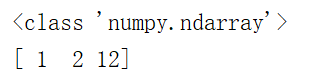3）mat x = mat([1,2,3]) y = mat([1,1,4]) mul = multiply(x,y) print(type(mul)) print(mul)
result：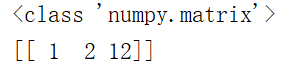4）mat，但x，y为行、列向量 x = mat([1,2,3]) y = mat([1,1,4]) mul = multiply(x,y.T) print(type(mul)) print(mul)
result：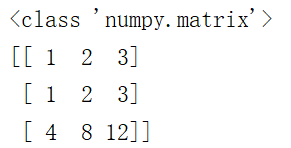运算 * ‘ * ’ ，与multiply类似，不过不能用于列表，mat形式为向量点乘 1）array x = array([1,2,3]) y = array([1,1,4]) mul = x*y print(type(mul)) print(mul)
result：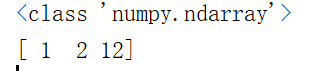2）mat，要求行列向量维度对齐（m×n * n×d = m×d） x = mat([1,2,3]) y = mat([1,1,4]) mul = x*y.T print(type(mul)) print(mul)
result：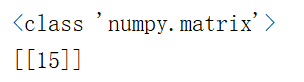dot（x，y）：为向量点乘 1）list x = [1,2,3] y = [1,1,4] mul = dot(x,y) print(type(mul)) print(mul)
result：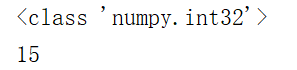2）array x = array([1,2,3]) y = array([1,1,4]) mul = dot(x,y) print(type(mul)) print(mul)
result：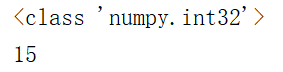3）mat x = mat([1,2,3]) y = mat([1,1,4]) mul = dot(x,y.T) print(type(mul)) print(mul)
result：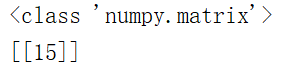总结：
multiply（x，y）为对应数字对应相乘，返回一个向量（或矩阵），对mat形式也不例外‘*’ ，mat形式的为点乘，其它为对应点相乘，list格式不能使用！dot（x，y）为点乘。
当mat格式为点乘时，要注意前一个的列与后一个的行对齐！
展开全文• Python代码 区别与联系 举例 总结 重点区别 点积与矩阵相乘的联系 前言 看“花书”的过程中碰到这样一句话 两个相同维数的向量x 和y 的点积（dot product）可看作是矩阵乘积x⊤y。 明明在讲矩阵相乘，...
目录
前言
向量
定义
与矩阵的关系
向量的乘法运算
矩阵
定义
矩阵乘积运算
Python代码
区别与联系
举例
总结
重点区别
点积与矩阵相乘的联系

前言
看“花书”的过程中碰到这样一句话

两个相同维数的向量x 和y 的点积（dot product）可看作是矩阵乘积x⊤y。

明明在讲矩阵相乘，怎么又扯到点积了？还有向量……
之前学得懵懵懂懂，为了深度学习，我仔细找资料写下这篇博客，送给与我一样情况的小伙伴。
PS:“花书”为图书AI圣经《深度学习》，由全球知名的三位专家 Ian Goodfellow、Yoshua Bengio 和 Aaron Courville联合撰写，是深度学习领域奠基性的经典教材。
向量
定义
向量是一列数。
举例：向量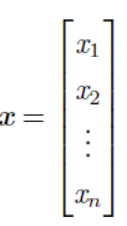与矩阵的关系
向量可以看作只有一列的矩阵
向量的转置可以看作是只有一行的矩阵
向量x的转置: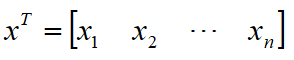向量的乘法运算
向量有很多运算，本文只说向量的乘法运算。
数量积（又叫内积、点积dot product; scalar product）

设二维空间内有两个向量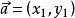和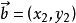，定义它们的数量积（又叫内积、点积）为以下实数：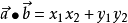更一般地，n维向量的内积定义如下：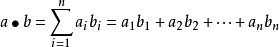参考百度百科

矩阵
定义
矩阵是一个二维数组
矩阵乘积运算
矩阵有很多运算，本文只说矩阵乘积运算。

设A为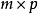的矩阵，B为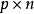的矩阵，那么称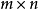的矩阵C为矩阵A与B的乘积，记作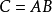，
其中矩阵C中的第行第列元素可以表示为：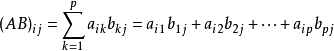矩阵相乘的前提条件：
矩阵A 的形状是，矩阵B 的形状是，C 的形状是
有两个矩阵A, B如下：
矩阵A的维数为3x2，矩阵B的维数为2x3，那么A、B相乘的结果矩阵C应该为3x3，其中m=3，p=2，n=3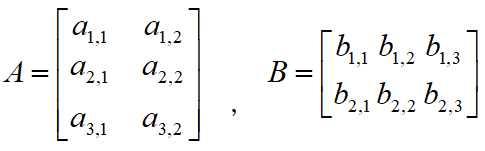根据公式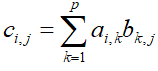，其中i, j取值范围为[1, 3], p=2
得出矩阵C各个元素为如下表格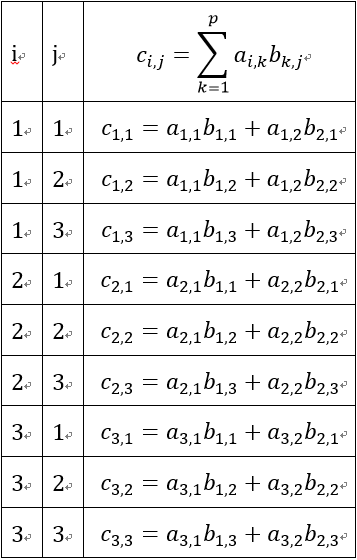即矩阵C为3x3的矩阵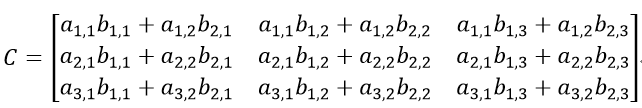简单地记：结果矩阵C的第(i, j)个元素为矩阵A的第 i 行与矩阵B的第 j 列分别相乘后求和的结果。
Python代码
写了一个简单的矩阵乘积方法，与np.dot(A, B, C)的结果是一样的。供参考。 以下方法的缺点是没法进行大数字的矩阵计算，比如A的维数为1000*10000，B的维数为10000*10000的情况.还需要再改进。
np.dot(A, B, C)也不能进行这么大数字的矩阵计算。
各位如果有更好的方法欢迎留言。
#! /usr/bin/env python
# -*- coding: utf-8 -*-

import numpy as np

def dot(A, B):
row = A.shape
column = B.shape
p = A.shape
if A.shape != B.shape:
return
# 创建一个矩阵C，维数为row*column, 其值全部为零
C = np.zeros((row, column), dtype=A.dtype)
print("A.shape, B.shape, C.shape:", A.shape, B.shape, C.shape)
# 计算矩阵相乘结果
for i in range(row):
for j in range(column):
for k in range(p):
C[i, j] += A[i, k] * B[k, j]
return C

if __name__ == '__main__':
A = np.arange(3, 9).reshape(2, 3)
B = np.arange(1, 7).reshape(3, 2)
C = np.zeros((2, 2), dtype=int)
print("matrix A:\n", A)
print("matrix B:\n",B)
np.dot(A, B, C)
print("np.dot C:\n", C)

c = dot(A, B)
print("my dot C:\n", c)
assert C.any() == c.any()
assert C.all() == c.all()
区别与联系

两个相同维数的向量x 和y 的点积（dot product）可看作是矩阵乘积x⊤y。

举例
以二维向量举例说明，这个比较简单好理解。二维看明白了就可以扩展地理解更多维数。
假设二维向量x和y分别为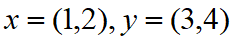x和y的点积（dot product）为如下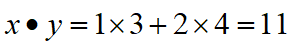将它们写成矩阵形式就是如下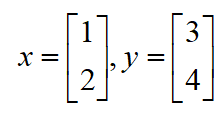矩阵x转置后为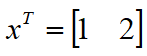，维数为1x2；矩阵y的维数为2x1；两个矩阵相乘，根据公式得到1x1的矩阵，如下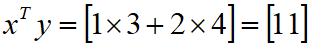敲黑板了！
总结
重点区别
两个向量点积结果是一个实数（即标量）
两个矩阵相乘结果是一个矩阵
重点：书上的原话中的“可看作” 不代表 "就是"
点积与矩阵相乘的联系
可以把矩阵乘积C = AB中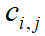看作是矩阵A 的第i 行和矩阵B 的第j 列之间的点积。
展开全文深度学习 花书 矩阵乘积
• 线性代数中两个向量相乘Prerequisite: Linear Algebra | Defining a Vector 先决条件： 线性代数| 定义向量 In the python code, we will add two vectors. We can add two vectors only and only if the both the...

线性代数中两个向量相乘
Prerequisite: Linear Algebra | Defining a Vector
先决条件： 线性代数| 定义向量
In the python code, we will add two vectors. We can add two vectors only and only if the both the vectors are in the same dimensional space. For our code, we consider the vectors in 4-dimensional space.
在python代码中，我们将添加两个向量。 仅当两个向量都在同一维空间中时，我们才可以添加两个向量。 对于我们的代码，我们考虑4维空间中的向量。
Python代码添加两个向量 (Python code to add two vectors)
#Vectors in Linear Algebra

a = [3, 5, -5, 8]
b = [4, 7, 9, -4]

print("Vector a = ", a)
print("Vector b = ", b)

# This is a 4 dimensional vector
# a list in python is a vector in linear algebra

sum = []
for i in range(len(a)):
sum.append(a[i] + b[i])

# This is how we can print a vector in python


Output
输出量
Vector a =  [3, 5, -5, 8]
Vector b =  [4, 7, 9, -4]
Vector Addition =  [7, 12, 4, 4]


线性代数中两个向量相乘

展开全文机器学习 线性代数 深度学习 算法
• A*B是矩阵（向量）对应元素相乘 np.dot(A,B)是矩阵乘法 A,B形式相同，各个元素相乘=B*A A,B满足矩阵相乘的条件。 A为2x2，B为1x2，所以是A的每一行和B各元素对应相乘 A为2x2，B为2x1，所以是A的每...
A*B是矩阵（向量）对应元素相乘
np.dot(A,B)是矩阵乘法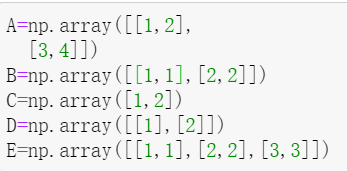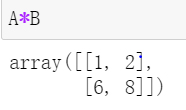A,B形式相同，各个元素相乘=B*A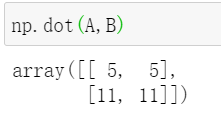A,B满足矩阵相乘的条件。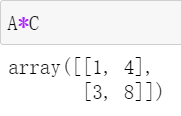A为2x2，B为1x2，所以是A的每一行和B各元素对应相乘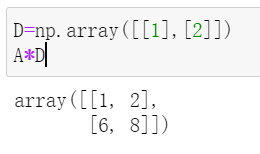A为2x2，B为2x1，所以是A的每一列和B各元素对应相乘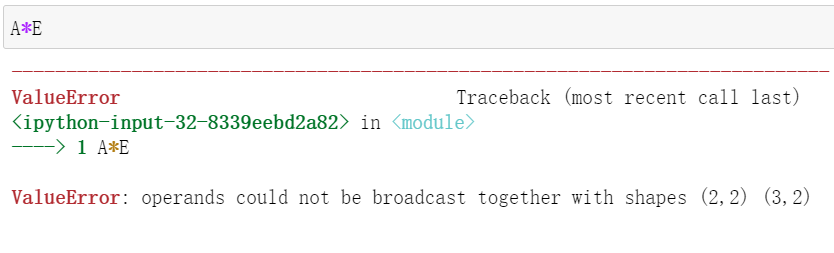A为2x2，E为3x2，没办法使对应元素相乘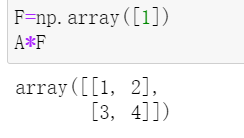F为1x1，对应元素相乘就直接用1*A的各个元素就可以

展开全文• Python中有时会碰到需要一个一维列向量（n*1）与另一个一维列向量（n*1）的转置（1*n）相乘，得到一个n*n的矩阵的情况。但是在python中，我们发现，无论是“.T”还是“np.transpose”都无法实现一维向量的转置，...
• python 向量交叉乘积与模计算 import numpy as np # 二维向量 交叉乘积 hxv = np.random.randint(1, 10, (3, 2), np.int32) hmv = np.random.randint(1, 10, (3, 2), np.int32) print('向量数组一\n', hxv) print('...线性代数 深度学习
• 计算：Ax-b A: 2*2 x: 2*1 b: 2*1 so, Ax-b: 2*1 if __name__ == &quot;__main__&quot;: A = np.array([[4.0, 1.0], [1.0, 3.0]]) b = np.array([[1.0], [2.0]]) x_0 = np.array([[2.0], [1...
• 假设有矩阵U= 和矩阵W = PS，必须保证这两个向量都是从numpy生成的 若要用U的第一列和W的第一行进行相乘，步骤如下： ...首先获取矩阵U的的第一列的列向量： ...最后，将列向量和行向量相乘 U[:,0].reshape...numpy
• 最近在熟悉python的科学计算，对于numpy的矩阵运算进行一些总结，和matlab还是很不一样的 import numpy as np 1、矩阵乘法 np.dot(a,b)，但a,b都为一维矩阵的时候，.dot实现内积，不用考虑a,b具体是行向量还是列...numpy 矩阵乘法
• 一、四元数乘法 定义： q=a+u⃗=a+bi+cj+dkq = a + \vec u = a + bi + cj + dkq=a+u=a+bi+cj+dk p=t+v⃗=t+xi+yj+zkp = t+\vec v = t + xi + yj + zkp=t+v=t+xi+yj+zk 运算法则 pq=at−u⃗⋅v⃗+av⃗+tu⃗u⃗×v⃗pq...
• 今天小编就为大家分享一篇解决Python计算矩阵乘向量,矩阵乘实数的一些小错误，具有很好的参考价值，希望对大家有所帮助。一起跟随小编过来看看吧
• numpy模块的array相乘时，有两种方式：一是矩阵形式，二是挨个相乘。 需要用矩阵形式相乘时，则要用np.dot()函数。 #矩阵与矩阵相乘a = np.array([[1,2,3],[4,5,6],[7,8,9]])c = a.copy()print(a * c)print(np.dot(a...
• 四元数与三维向量相乘运算法则 参考网站： https://www.cnblogs.com/jeason1997/p/9822353.html 四元数和向量相乘可以表示这个向量按照这个四元数进行旋转之后得到的新的向量。 例如：向量 c(0,0,10) 绕着Y轴旋转90...
• 矩阵相乘： 输入两个整数类型的矩阵mat1（m行d列）和mat2（d行n列），返回矩阵相乘后的结果mat1*mat2（m行n列）。矩阵均用二维列表进行表示。 def func3(m1, m2): m = len(m1) n = len(m2) m3 = [[0 for i ...
• 主要介绍了Python实现的矩阵转置与矩阵相乘运算,结合实例形式分析了Python针对矩阵进行转置与相乘运算的相关实现技巧与操作注意事项,需要的朋友可以参考下
• 向量点乘和对应分量相乘： >>> a array([1, 2, 3]) >>> b array([ 1., 1., 1.]) >>> np.multiply(a,b) array([ 1., 2., 3.]) >>> np.dot(a,b) 6.0 矩阵乘法和对应分量相乘： >>> c matrix([[1, 2, 3]]) >>> d matrix...
• 今天小编就为大家分享一篇Python 实现两个列表里元素对应相乘的方法，具有很好的参考价值，希望对大家有所帮助。一起跟随小编过来看看吧
• 线性代数向量乘法Prerequisite: Linear Algebra | Defining a Vector 先决条件： 线性代数| 定义向量 Linear algebra is the branch of mathematics concerning linear equations by using vector spaces and ...线性代数 机器学习 神经网络 算法
• Python的向量和矩阵乘法意义...#python 矩阵和向量相乘的意义 #Author:sw #Time:2020/10/10 import numpy as np #向量与向量相乘 a = np.array([1,2]) #为行向量(1,2) shapes(2,) b = np.array([1,2,3])#为行向量(1,2算法 人工智能 机器学习 线性代数
• ## np.dot两个向量相乘

千次阅读 2018-04-19 22:42:40
python里用numpy在进行向量相乘时，希望两个向量相乘得到矩阵形式。这里需要对没个向量增加一个维度才能相乘得到矩阵。python代码如下：import numpy as np a = np.array([1, 2, 3]) # shape: (3,) b = np.array([1,...
• ## python对应位置相乘

千次阅读 2019-05-28 13:32:14
import time import numpy as np A = np.arange(1,1000001).reshape(500,1000,2) B = np.arange(0,1000000).reshape(500,1000,2) for i in range(10): start=time.time() np.multiply(A,B) ... p...
• #定义：向量化计算是一种特殊的并行计算的方式，它可以在同一时间执行多次操作，通常是对不同的数据执行同样的一个或一批指令，或者说把指令应用于一个数组/向量。 #1. 四则运算 #规则：相同位置的数据进行运算...
• python中，数组和矩阵相乘与我们在线型代数中将向量看成一个列向量的思路是非常不同的，在python中数组的储存是按行存储的（在C语言，matlab中也是按行存储）。 根据吴恩达老师的建议，他在搭建神经网络时，一般...数组 矩阵
• 1、向量、矩阵表示： numpy的向量表示是通过array数组来实现的 一维向量（行、列）： 在numpy中一维向量用一位数组array([1, 1, 1])表示，既能表示行向量也能表示列向量，一维向量转置后还是原来的样子（因为存储...array matrix
• 手动实现法 # coding:utf-8 import numpy as np A = np.asarray([[1,2,3],[4,5,6],[7,8,9]]) B = np.asarray([[2,3],[1,2],[6,7]... print("两个矩阵无法相乘") else: # 时间复杂度为：A:m*n B:n*k 则为O(m*k*n) r.算法
• 2019独角兽企业重金招聘Python工程师标准>>> ...
• python求两个向量的夹角 import numpy as np x=np.array([3,5]) y=np.array([4,2]) # 两个向量 Lx=np.sqrt(x.dot(x)) Ly=np.sqrt(y.dot(y)) #相当于勾股定理，求得斜线的长度 cos_angle=x.dot(y)/(Lx*Ly) #求得cos_...
• numpy 向量乘法Prerequisite: Defining Vector using Numpy 先决条件： 使用Numpy定义Vector To read about the Scalar Multiplication of Vector, visit: Scalar Multiplication of Vector 要了解向量的标量乘法...numpy 机器学习 线性代数 深度学习...

# python向量相乘python 订阅